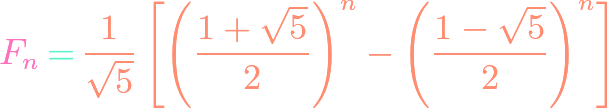## Binet’s Formula for Fibonacci Numbers

https://www.youtube.com/watch?v=bgjQDilbHE8 The Fibonacci numbers are among the most prolific of number sequences, perhaps second only to the Catalan numbers. They can be defined recursively, but one may ask if there exists a closed formula for the sequence. This is answered by Binet’s formula, which takes on a form so strange that it is hard to …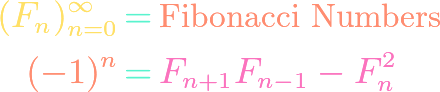## Cassini’s Identity for Fibonacci Numbers

https://www.youtube.com/watch?v=WED3oFewP-4 The Fibonacci numbers are among the most famous of number sequences. They satisfy a plethora of identities, including Cassini’s identity. Cassini is not easy to prove by elementary means, but an ingenious trick via matrix multiplication and the mutliplicativity of the determinant does the job. We provide this proof in this video.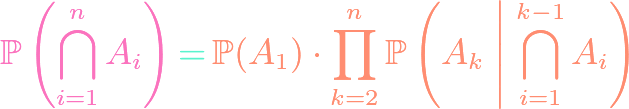## Chain Rule for Events in Probability

https://www.youtube.com/watch?v=6LPf6OleUFA Conditional probability is a tricky but useful concept. One of the most useful tools in conditional probability is the chain rule, which allows us to decompose the probability of the intersection of a finite number of events as the product of some conditional probabilities. We state and prove the chain rule for probability in …## Classifying the Platonic Solids

https://www.youtube.com/watch?v=sgXXcwdn5p8 The Platonic solids are regular polyhedra, meaning each vertex has the same number of edges incident on it, and each face has the same number of edges on its border. It is known that there exist five Platonic solids: the tetrahedron, cube, octahedron, dodecahedron, and icosahedron. By using Euler’s formula for planar graphs, and …## A Criterion for Planar Graphs

https://www.youtube.com/watch?v=be8AKUAAuZs It is difficult to know in general when a graph has a planar embedding without actually seeing a planar embedding. However, we can derive a necessary criterion for planarity using Euler’s characteristic formula, depending only on the number of vertices and edges of the graph (making it independent of any particular planar embedding). By …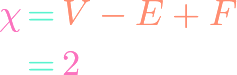## Euler’s Characteristic Formula for Planar Graphs

https://www.youtube.com/watch?v=_qfNDM1X53U Euler’s formula tells us that, for any planar embedding of a connected planar graph, the number of vertices minus the number of edges plus the number of faces (including the unbounded face) is always $2$. This amazing fact is proven in our video by induction. A part of the argument is to do casework …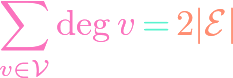## Handshaking Lemma in Graph Theory

https://www.youtube.com/watch?v=8siY3EjTXxE Sometimes called the first theorem of graph theory, the handshaking lemma consists of a main lemma and a consequent corollary. The first part tell us that in a finite, simple graph the sum of the degrees of the vertices equals two times the total number of edges. The second part tells us that the …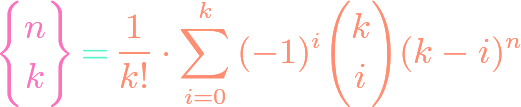## Stirling Partition Number of a Set

https://www.youtube.com/watch?v=PFJNugCr9_I Stirling numbers of the second kind count the number of ways of partitioning a set of $n$ elements into $k$ non-empty disjoint subsets whose union is the original set. This is equivalent to counting the number of ways of distributing $n$ distinguishable balls into $k$ indistinguishable boxes. The problem turns out to reduce to …## Balls & Urns, Sticks & Stones, Stars & Bars

https://www.youtube.com/watch?v=lcjNoFJ3Ick In how many ways can $n$ indistinguishable balls be placed into $k$ distinguishable boxes? What if each box must receive at least one ball? It turns out that the answers can be expressed as binomial coefficients. However, the standard journey to finding them requires an ingenious trick. We discuss and apply the technique in …